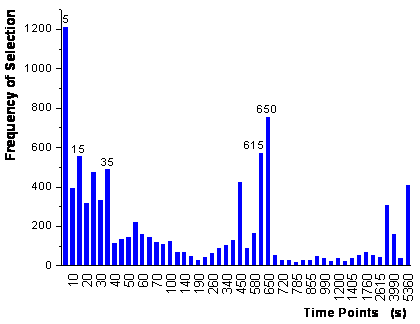Home News About Me Ph. D. Thesis Abstract Table of Contents 1. Introduction 2. Theory  Fundamentals of the Multivariate Data Analysis 3. Theory  Quantification of the Refrigerants R22 and R134a: Part I 4. Experiments, Setups and Data Sets 5. Results  Kinetic Measurements 6. Results  Multivariate Calibrations 7. Results  Genetic Algorithm Framework 8. Results  Growing Neural Network Framework 9. Results  All Data Sets 9.1. Methanol and Ethanol by SPR 9.2. Methanol, Ethanol and 1-Propanol by SPR 9.2.1. Single Analytes 9.2.2. Multivariate Calibrations of the Mixtures 9.2.3. Genetic Algorithm Framework 9.2.4. Parallel Growing Neural Network Framework 9.2.5. PCA-NN 9.2.6. Conclusions 9.3. Methanol, Ethanol and 1-Propanol by the RIfS Array and the 4l Setup 9.4. Quaternary Mixtures by the SPR Setup and the RIfS Array 9.5. Quantification of the Refrigerants R22 and R134a in Mixtures: Part II 10. Results  Various Aspects of the Frameworks and Measurements 11. Summary and Outlook 12. References 13. Acknowledgements Publications Research Tutorials Downloads and Links Contact Search Site Map Print this Page9.2.4.   Parallel Growing Neural Network Framework

Also, the parallel growing neural network framework introduced in chapter 8 was applied to the data with 500 parallel runs of the growing neural networks for each analyte. The ranking of the variables, which is combined for all analytes similar to section 9.1.2, is shown in figure 66. The second step of the algorithm (20-fold random subsampling sets) stopped after the addition of 5 variables, which are labeled in figure 66. Compared with the variable selection by the GA framework, the selection by the parallel growing network framework looks similar, but not identical. Instead of the signal at 20 s the time point 35 s is used (variation of ethanol and 1-propanol) and instead of the time point 55 s the signal at 650 s is used (main variation of 1-propanol). According to table 6, the corresponding optimized neural networks (4 hidden neurons and 1 output neuron) showed the best predictions of all methods used for this data set.figure 66:  Frequency of the variables selected in the first step of the parallel growing neural network framework

The randomization test (200 parallel runs of the growing nets) demonstrates that the parallel growing neural network framework is highly reproducible with the selection of the same 5 variables (see figure 67). When comparing figure 67 with figure 65, it is obvious that the growing neural nets are less subject to selecting random variables due to chance correlation than the genetic algorithm.figure 67:  Ranking of the variables for 50 time points and for 50 additional random variables.

 Page 124 © Frank Dieterle, 03.03.2019